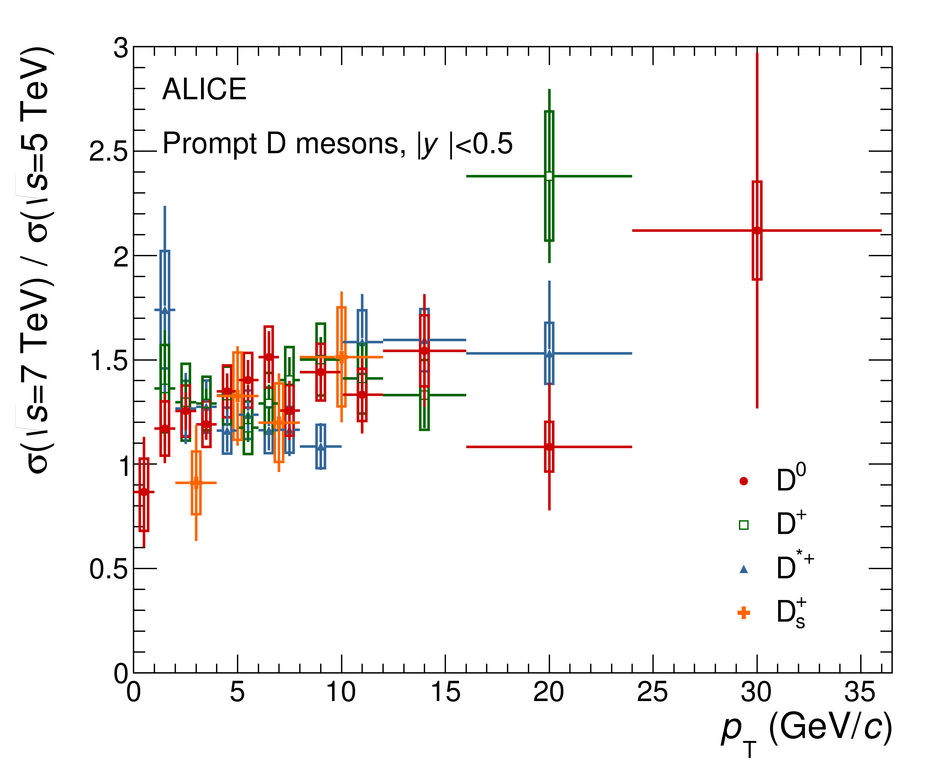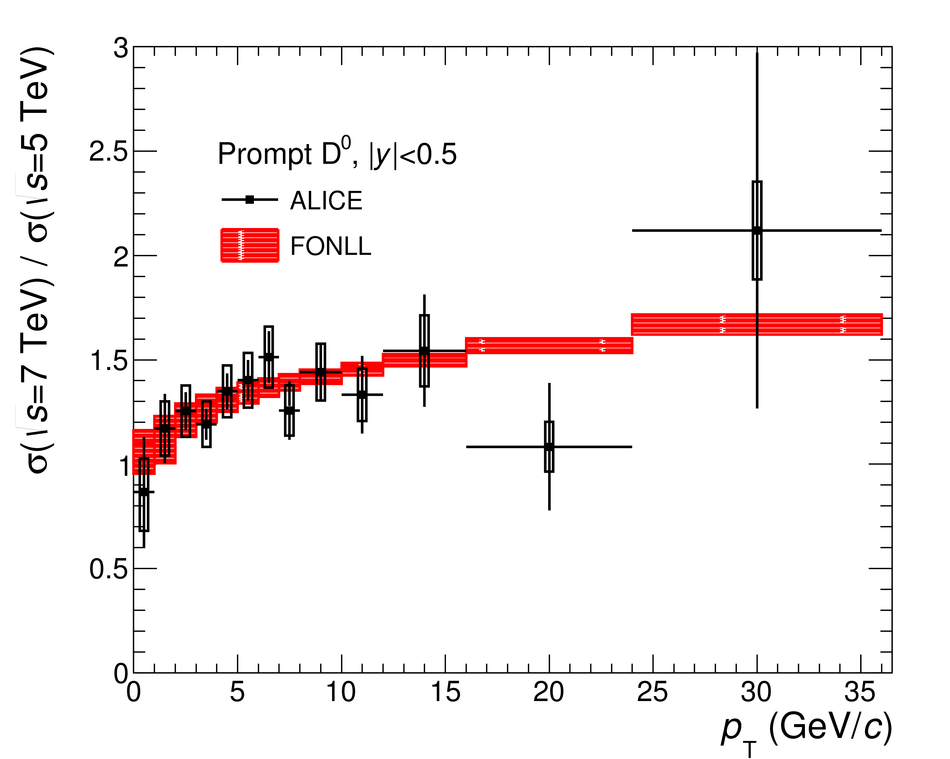# Figure 14

 Ratios of $\Dzero$, $\Dplus$, $\Dstar$ and $\Ds$-meson production cross sections in pp collisions at $\sqrts=7$~TeV~ and $\sqrts=5.02$~TeV as a function of $\pt$ (left panel). $\Dzero$ ratio compared to FONLL pQCD calculations~ (right panel).